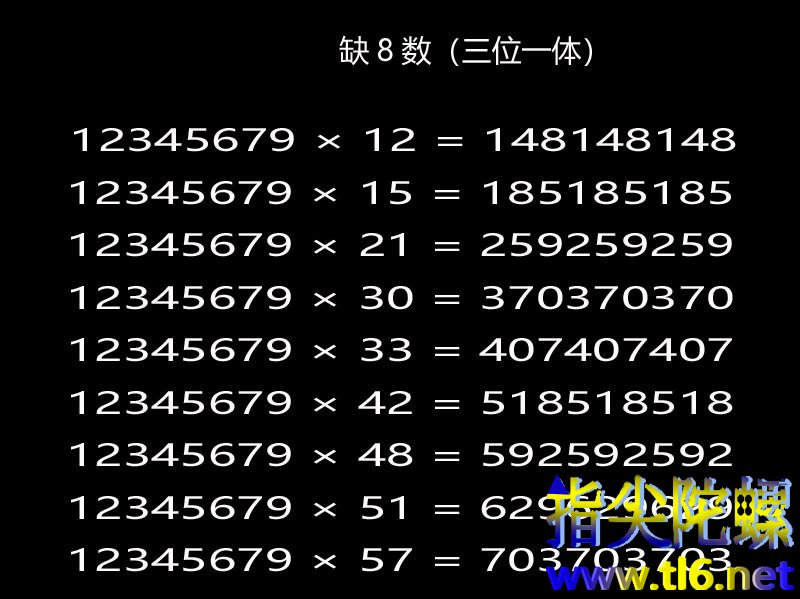＂缺８数＂——１２３４５６７９,颇为神秘,故许多人在进行探索．

＂缺８数＂实际上并非对７情有独钟,它是＂一碗水端平＂,对所有的数都＂一视同仁＂的:你只要分别用９的倍数（９,１８......直到８１）去乘它,则１１１１１１１１１,２２２２２２２２２......直到９９９９９９９９９都会相继出现．

１２３４５６７９×１２=１４８１４８１４８

１２３４５６７９×１５=１８５１８５１８５

１２３４５６７９×５７=７０３７０３７０３

１２３４５６７９×１０=１２３４５６７９０（缺８）

１２３４５６７９×１１=１３５８０２４６９（缺７）

１２３４５６７９×１３=１６０４９３８２７（缺５）

１２３４５６７９×１４=１７２８６９５０６（缺４）

１２３４５６７９×１６=１９７５３０８６４（缺２）

１２３４５６７９×１７=２０９８７６５４３（缺１）

（１）乘数为９的倍数

１２３４５６７９×２４３=２９９９９９９９９７,只要把乘积中最左边的一个数２加到最右边的７上,仍呈现＂清一色＂．（２）乘数为３的倍数,但不是９的倍数

１２３４５６７９×８４=１０３７０３７０３６,只要把乘积中最左边的一个数１加到最右边的６上,又可看到＂三位一体＂现象．（３）乘数为３k+１或３k+２型

１２３４５６７９×９８=１２０９８７６５４２,表面上看來,乘积中出现雷同的２,但据上所說,只要把乘积中最左边的数１加到最右边的２上去之后,所得数为２０９８７６５４３,是＂缺１＂数,而根据上面的＂学说＂可知,此时正好轮到１休息,结果与理论完全吻合．

１２３４５６７９×２８=３４５６７９０１２

１２３４５６７９×３７=４５６７９０１２３

１２３４５６７９×４=４９３８２７１６

１２３４５６７９×５=６１７２８３９５

１２３４５６７９×６７=８２７１６０４９３

１２３４５６７９×６８=８３９５０６１７２